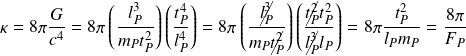# Einstein gravitational constant

The Einstein gravitational constantappears in the Einstein Field Equations.

The constant gives the maximum force potential while the stress-energy tensoracts as a proportionality operator reducing the maximum potential in the right proportions to produce the gravitational force.

The Einstein gravitational constant is defined by the following relationship between the composite gravitational constant and the speed of light.

Restating the gravitational constant in fundamental units gives the following simple form of the Einstein gravitational constant

whereis the Planck force. The constant is derived by replacingwith fundamental Planck units.

The elementary form of the Einstein gravitational constant is

Einstein gravitational constant